Tutorial #03: Pong

In this tutorial we will use the knowledge gained from the previous tutorials and create a simple game after the model of Atari's Pong. The game will be refined and adapted for smart phones and tablets within the subsequent tutorials.

# Version 1: Paddle and Ball

We start by defining the graph nodes in the XML files. As a result, the screen should now display a rectangle on the right and left side for the paddles, a square in the center for the ball and a vertical dashed midline for the net.

## Exercises

• Try to first develop a version on your own and compare the result with the solution below afterwards.

For displaying the ball and the paddles we use `PlaneGeometry` nodes and scale them with `scaleFactorX` and `scaleFactorY` to the desired size.

```<?xml version="1.0" ?>

<Graph>
<Instance graphResourceId="package_main:graph_mat"/>

<View id="view"/>
<Camera
id="camera"
viewId="view"
fieldOfViewX="400"
nearPlane="400" farPlane="2500"
clearColorBuffer="1"
/>
<CameraTransform
cameraId="camera"
posX="0" posY="0" posZ="800"
/>
<CameraState
cameraId="camera"
/>

<MaterialState
materialId="material/mat_white"
/>

<PlaneGeometry
scaleFactorX="20"
scaleFactorY="100"
posX="-350" posY="0" posZ="0"
/>

<PlaneGeometry
scaleFactorX="20"
scaleFactorY="100"
posX="350" posY="0" posZ="0"
/>

<PlaneGeometry
id="ball"
scaleFactor="10"
posX="0" posY="0" posZ="0"
/>
```

The dashed midline is created with fourteen individual dashes. Normally, we would have to define fourteen `PlaneGeometry` elements in the XML file. For reasons of convenience, it is also possible to use the `replications` attribute of a `Graph::Instance` node in order to repeatedly instantiate an object. By specifying `replications="14"`, the resource with the given ID is instantiated fourteen times, and each of these instances is added to the graph:

```    <Instance graphResourceId="package_main:graph_line" replications="14" myWidth="5"/>
</Graph>
```

In order to keep a difference between the `id`-names of the instances, the resource file receives the attribute replication with the instance number as value. Within the resource file, every `"{replication}"` will be replaced with its corresponding instance index, ranging from 0 to (replications-1).

```<?xml version="1.0" ?>

<Graph myWidth="5">
<PlaneGeometry
id="line_{replication}"
scaleFactorX="{myWidth}"
scaleFactorY="20"
posX="0" posY="400" posZ="0"
/>
</Graph>
```

As a result, the IDs of the created instances are `"line_0"`, `"line_1"`, `"line_2"` etc., up to `"line_13"`.

Following the same scheme, at the `Instance` node it is also possible to define custom attributes and to pass them to the resource file. For example, the width of the line elements can be passed as a custom attribute named `"myWidth"` with a defined value, e.g. `myWidth="2"`. Within the resource file, every occurrence of `{myWidth}` is replaced by the given value:

```    <Instance graphResourceId="package_main:graph_line" replications="14" myWidth="2"/>
```

In the corresponding graph resource file, default values can be specified for custom attributes in the root element `Graph`. This prevents values from remaining undetected, if their specification has been forgotten when creating the instance. The value of the instance overwrites the default value:

```<?xml version="1.0" ?>

<Graph myWidth="5">
<PlaneGeometry
id="line_{replication}"
scaleFactorX="{myWidth}"
scaleFactorY="20"
posX="0" posY="400" posZ="0"
/>
</Graph>
```
Note
Passing attribute values with attribute names put in braces is a special feature of the Murl engine and not conformant to XML standards.

In the file `pong_logic.h` we define three `TransformNode` member objects in order to manipulate the paddles and the ball:

```            Logic::TransformNode mBallTransform;
```

Again, the node elements are linked in the `OnInit()` method.

```Bool App::PongLogic::OnInit(const Logic::IState* state)
{

Graph::IRoot* root = state->GetGraphRoot();
//root->PrintTree();
```

The `TransformNode` `mBallTransform` is used to define the Y-axis of the fourteen line elements. For every line element a reference is created. As soon as the position is set, the reference is released.

```    // lay out middle line
SInt32 i;
const SInt32 maxInstances = 14;
for (i=0; i < maxInstances; i++)
{
mBallTransform.GetReference(root, "line_"+Util::UInt32ToString(i));
mBallTransform->SetPositionY(-20.0 + (maxInstances/2 - i)*40);
mBallTransform.RemoveReference();
}
```

Afterwards, a connection between the scene graph nodes for paddles and ball and their respective logic member objects is created by using the `TransformNode::GetReference()` and `BaseProcessor::AddGraphNode()` methods:

```    // get references for ball and paddles
if (!AreGraphNodesValid())
{
return false;
}

return true;
}
```

As a result, we get the pong playfield with two paddles, one ball and a dashed midline: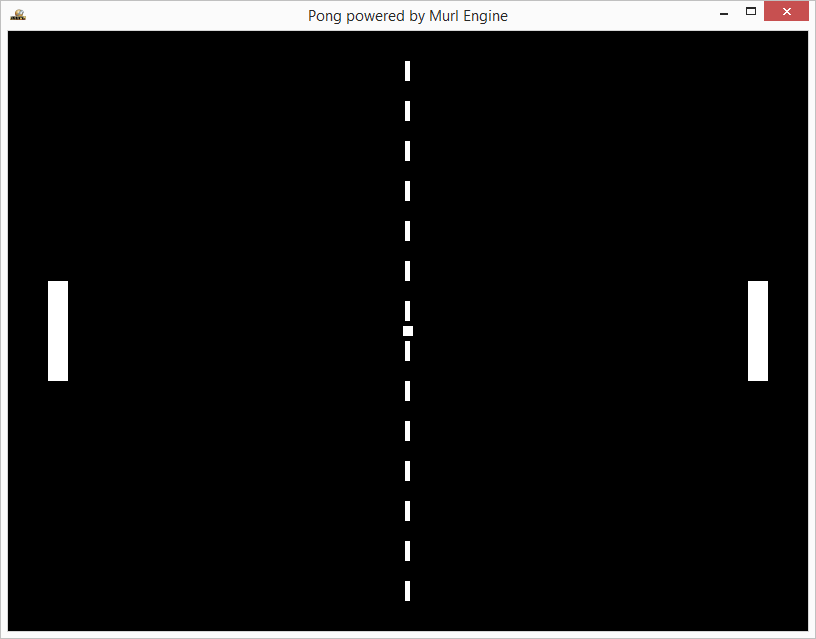Pong V1 output window showing the playfield

# Version 2: Controls

As a next step, the code is extended in order to control the paddles and the ball. For now, we will be able to control the paddles only via keyboard and mouse.

We declare a new method to update the position of the paddles and two new member variables to save their current position:

```            void UpdatePaddlePosition(Logic::IDeviceHandler* deviceHandler, Double tickDuration);
```
```            Real mPaddleLeftPosY;
```

Both member variables are initialized to 0 in the constructor:

```App::PongLogic::PongLogic(Logic::IFactory* factory)
: BaseProcessor(factory)
, mGameIsPaused(true)
, mBallPosX(0)
, mBallPosY(0)
, mBallDirectionX(1)
, mBallDirectionY(1)
, mBallSpeed(1)
{
}
```

The new method is then called in the `OnProcessTick()` method. The `deviceHandler` and `tickDuration` are passed as parameters.

By using the `deviceHandler`, inputs from e.g. touch pad, mouse or keyboard can be queried. The value in the variable `tickDuration` is exactly the tick time indicated in seconds, which will pass till the next call of `OnProcessTick()` method. With this value, the exact distance covered by the ball and its new position can be calculated, if a certain speed has been set.

```void App::PongLogic::OnProcessTick(const Logic::IState* state)
{
Logic::IDeviceHandler* deviceHandler = state->GetDeviceHandler();
Double tickDuration = state->GetCurrentTickDuration();

}
```

The method `UpdatePaddlePosition()` determines the position of the paddle. For the right paddle we use the method `IDeviceHandler::GetMousePosition()`, which indicates the position of the mouse cursor. The determined X and Y values always range between -1 and +1 being therefore independent of window size, aspect ratio or camera angle.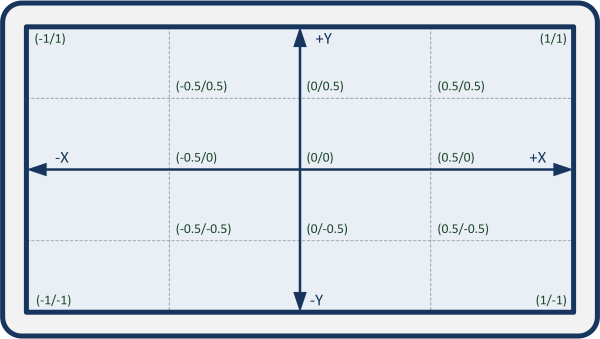Mouse coordinate input range

The virtual world shown has a size of 800 x 600 units on the Z-level 0. Therefore, we need to multiply the Y value by 300 in order to convert the Y value of the mouse coordinate into a Y value of the virtual world.

```void App::PongLogic::UpdatePaddlePosition(Logic::IDeviceHandler* deviceHandler, Double tickDuration)
{
if (deviceHandler->WasMouseMoved())
{
Real posX;
}
```

For the left paddle we use the method `IDeviceHandler::IsRawKeyPressed()`. The step size is multiplied by the `tickDuration` in order to get a constant speed for the paddle independent of the frame rate. Otherwise, the paddle would move at a different speed dependent of the logic step size.

```    // Left Paddle Keyboard
if (deviceHandler->IsRawKeyPressed(RAWKEY_UP_ARROW ))
{
}
if (deviceHandler->IsRawKeyPressed(RAWKEY_DOWN_ARROW ))
{
}
}
```

## Game States

Following the same scheme we declare a new member variable `mGameIsPaused` and a protected `UpdateGameStates()` method in order to switch between individual game states.

```void App::PongLogic::OnProcessTick(const Logic::IState* state)
{
Logic::IDeviceHandler* deviceHandler = state->GetDeviceHandler();
Double tickDuration = state->GetCurrentTickDuration();

UpdateGameStates(deviceHandler);
}
```

We use the left mouse button or the space bar to pause the game and the ESC key or back button to close the application.

```void App::PongLogic::UpdateGameStates(Logic::IDeviceHandler* deviceHandler)
{
// Game Pause
if ((deviceHandler->WasRawKeyPressed(RAWKEY_SPACE)) ||
(deviceHandler->WasMouseButtonPressed(IEnums::MOUSE_BUTTON_LEFT)))
{
mGameIsPaused = !mGameIsPaused;
}

// Exit
if (deviceHandler->WasRawKeyPressed(RAWKEY_ESCAPE) ||
deviceHandler->WasRawButtonPressed(RAWBUTTON_BACK))
{
deviceHandler->TerminateApp();
}
}
```

## Ball Position

In order to calculate the movement of the ball, we declare several methods and member variables.

```            void InitBallPosition();
void MissedBall();
void SetAndNormalizeBallDirection(Real x, Real y);
void UpdateGameStates(Logic::IDeviceHandler* deviceHandler);
void UpdateBallPosition(Logic::IDeviceHandler* deviceHandler, Double tickDuration);

Logic::TransformNode mBallTransform;

Util::TT800 mRandomNumberGenerator;

Bool mGameIsPaused;
Real mBallPosX, mBallPosY;
Real mBallDirectionX, mBallDirectionY;
Real mBallSpeed;
```

The method `SetAndNormalizeBallDirection()` sets a new value for the direction of the ball and normalizes the length of the direction vector to 1. By doing so, the ball speed can be set independently of the direction vector.

```void App::PongLogic::SetAndNormalizeBallDirection(Real x, Real y)
{
// Normalize and update direction vector
Real divisor = Math::Sqrt(x*x + y*y);
x /= divisor;
y /= divisor;
mBallDirectionX = x;
mBallDirectionY = y;
}
```

The method `InitBallPosition()` is used for the initialization of the values. In order to randomly set the direction of the ball, an instance (`mRandomNumberGenerator`) of the random number generator class `Util::TT800` is used. The method `Murl::Util::Rng::RandReal(Real,Real),RandReal(Real(-1.0), Real(+1.0))` determines a random value between -1 and +1.

```void App::PongLogic::InitBallPosition()
{
Real randomNumber = mRandomNumberGenerator.RandReal(Real(-1.0), Real(+1.0));

mBallPosX = 0;
mBallPosY = 0;
SetAndNormalizeBallDirection(Real(1), randomNumber);
mBallSpeed = 400;
mBallTransform->SetPositionX(mBallPosX);
mBallTransform->SetPositionY(mBallPosY);
mGameIsPaused = true;
}
```

The current position of the ball is calculated through the method `UpdateBallPosition()`. If the ball misses one of the paddles the method `MissedBall()` is called. Depending on where the ball hits the paddle, its direction changes when it is reflected. Furthermore, the speed of the ball slightly increases with every successful setback.

```void App::PongLogic::UpdateBallPosition(Logic::IDeviceHandler* deviceHandler, Double tickDuration)
{
if (mGameIsPaused)
return;

mBallPosX += mBallDirectionX*mBallSpeed*tickDuration;
mBallPosY += mBallDirectionY*mBallSpeed*tickDuration;

// Intentional ignore ball width for collisionPosition
// collisionPositionX = paddlePosition - paddleWidth/2 = 350 - 20/2 = 340
if (mBallPosX >= 340)
{
Real distance = mPaddleRightPosY*300 - mBallPosY;
if (Math::Abs(distance) <= 55)
{
SetAndNormalizeBallDirection(Real(-1),Real(-2)*distance/55);
mBallSpeed += Real(50);
}
else
{
MissedBall();
}
}

// Intentional ignore ball width for collisionPosition
if (mBallPosX <= -340)
{
Real distance = mPaddleLeftPosY*300 - mBallPosY;
if (Math::Abs(distance) <= 55)
{
SetAndNormalizeBallDirection(Real(1), Real(-2)*distance/55);
mBallSpeed += Real(50);
}
else
{
MissedBall();
}
}

if (mBallPosY > 295)
{
mBallPosY = 590 - mBallPosY;
mBallDirectionY *= -1;
}
else if (mBallPosY < -295)
{
mBallPosY = -590 - mBallPosY;
mBallDirectionY *= -1;
}

mBallTransform->SetPositionX(mBallPosX);
mBallTransform->SetPositionY(mBallPosY);
}
```

In the method `MissedBall()` the variables are initialized and the direction of the ball is corrected to the effect that after restarting the game it always starts towards the opposite paddle to score a point.

```void App::PongLogic::MissedBall()
{
if (mBallPosX > 0)
{
InitBallPosition();
mBallDirectionX *= -1;
}
else
{
InitBallPosition();
}
}
```

The result is a first playable version of the game, but so far without score counter.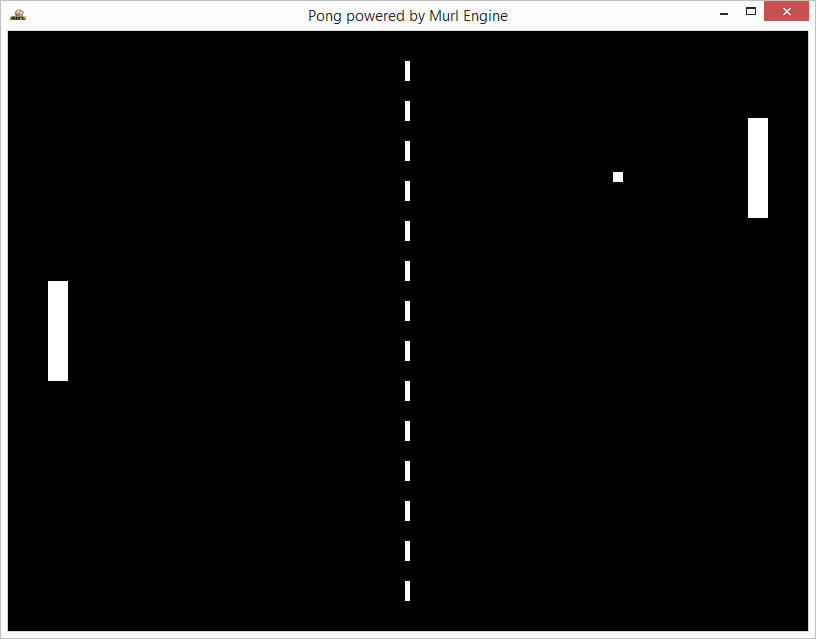Pong V2 output window

# Version 3: Score Counter

Finally, we create a one-digit indicator to display score points and errors.

```<?xml version="1.0" ?>

<Graph posX="0" posY="0" idExt="">
<Namespace id="segment{idExt}">
<Transform
id="transform"
posX="{posX}" posY="{posY}"
>
<PlaneGeometry
id="a"
scaleFactorX="56"
scaleFactorY="6"
posX="0" posY="50" posZ="0"
/>
<PlaneGeometry
id="b"
scaleFactorX="6"
scaleFactorY="53"
posX="25" posY="25" posZ="0"
/>
<PlaneGeometry
id="c"
scaleFactorX="6"
scaleFactorY="53"
posX="25" posY="-25" posZ="0"
/>
<PlaneGeometry
id="d"
scaleFactorX="56"
scaleFactorY="6"
posX="0" posY="-50" posZ="0"
/>
<PlaneGeometry
id="e"
scaleFactorX="6"
scaleFactorY="53"
posX="-25" posY="-25" posZ="0"
/>
<PlaneGeometry
id="f"
scaleFactorX="6"
scaleFactorY="53"
posX="-25" posY="25" posZ="0"
/>
<PlaneGeometry
id="g"
scaleFactorX="56"
scaleFactorY="6"
posX="0" posY="0" posZ="0"
/>
</Transform>
</Namespace>
</Graph>
```

We create a score counter for the left and the right player each.

```    <Instance graphResourceId="package_main:graph_line" replications="14" myWidth="5"/>
<Instance graphResourceId="package_main:graph_segment" posX="-180" posY="200" idExt="Left"/>
<Instance graphResourceId="package_main:graph_segment" posX="180" posY="200" idExt="Right"/>
```

In the file `pong_logic.h` we declare a variable for the score for each player, a `TransformNode` to save the reference to the score node as well as the methods `ResetScore()` and `UpdateScore()`.

```            void ResetScore();
UInt32 scoreLeft, scoreRight;
Logic::TransformNode mSegmentLeftTransform;
Logic::TransformNode mSegmentRightTransform;
```

The method `OnInit()` allows saving the transform node with the ID `"transform"`. The namespaces are `"segmentLeft"` and `"segmentRight"` .

```    AddGraphNode(mSegmentLeftTransform.GetReference(root, "segmentLeft/transform"));
```

In the method `ResetScore()` both of the score values are set to 0. Furthermore, the method `UpdateScore()` is called.

```void App::PongLogic::ResetScore()
{
scoreLeft = 0;
scoreRight = 0;
}
```

With the method `UpdateScore()` it is possible to make the segments visible or invisible depending on the score value.

At first, the method `ITransform::GetNodeInterface()` retrieves a pointer to the transform node's `Graph::INode` interface. Afterwards, the method call `node->GetChild(i)` successively accesses the child nodes. The coding for the individual segments is fixed with the array `displayValues` from 0 to 9. The low-order bit controls segment a, the next bit segment b etc.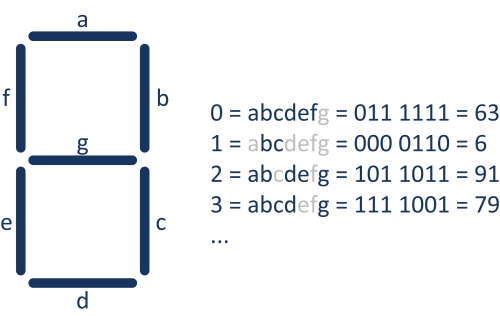The seven segments of our score counter

The modulo operator `score % 10` ensures that the array access is limited to the value range 0 to 9. With `displayValue % 2` the low-order bit is masked out and `displayValue >> 1` shifts the value by one bit to the right (i.e. a division by 2).

```void App::PongLogic::UpdateScore(Logic::TransformNode& transform, UInt32 score)
{
Graph::INode* node = transform->GetNodeInterface();

const UInt32 displayValues = {63, 6, 91, 79, 102, 109, 125, 7, 127, 111};
int displayValue = displayValues[score % 10];

UInt32 i;
for (i=0; i<7; i++)
{
if ((displayValue % 2) == 1)
node->GetChild(i)->SetVisible(true);
else
node->GetChild(i)->SetVisible(false);
displayValue = displayValue >> 1;
}
}
```

As a last step, we adjust the method `UpdateGameStates()`. If the maximum error score of 9 has been reached, a new game should start, setting the score of both players to 0.

```void App::PongLogic::UpdateGameStates(Logic::IDeviceHandler* deviceHandler)
{
// Game Pause
if ((deviceHandler->WasRawKeyPressed(RAWKEY_SPACE)) ||
(deviceHandler->WasMouseButtonPressed(IEnums::MOUSE_BUTTON_LEFT)))
{
mGameIsPaused = !mGameIsPaused;

if (mGameIsPaused == false)
if ((scoreLeft == 9) || (scoreRight == 9))
ResetScore();
}

// Exit
if (deviceHandler->WasRawKeyPressed(RAWKEY_ESCAPE) ||
deviceHandler->WasRawButtonPressed(RAWBUTTON_BACK))
{
deviceHandler->TerminateApp();
}
}
```

As a result, we receive a playable version of the classic game Pong.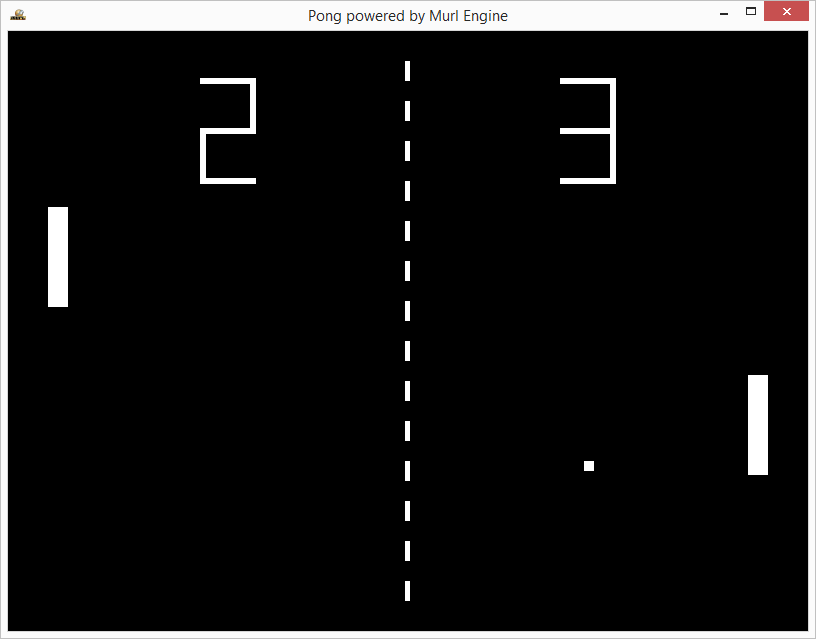Pong V3 output window